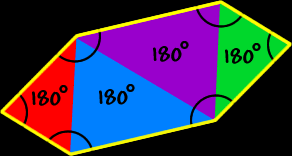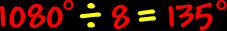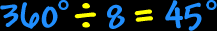# Polygons - Octagons

Properties of octagons, interior angles of octagons

Polygons:  Properties of OctagonsSum of the Interior Angles of an Octagon:
 This image shows the process for a HEXAGON:Using the same methods as for hexagons to the right (I'll let you do the pictures)... To find the sum of the interior angles of an octagon, divide it up into triangles... There are six triangles...  Because the sum of the angles of each triangle is 180 degrees...  We getSo, the sum of the interior angles of an octagon is 1080 degrees.Regular Octagons:
The properties of regular octagons:All sides are the same length (congruent) and all interior angles are the same size (congruent).

To find the measure of the angles, we know that the sum of all the angles is 1080 degrees (from above)...  And there are eight angles...So, the measure of the interior angle of a regular octagon is 135 degrees.The measure of the central angles of a regular octagon:To find the measure of the central angle of a regular octagon, make a circle in the middle...  A circle is 360 degrees around...  Divide that by eight angles...So, the measure of the central angle of a regular octagon is 45 degrees.